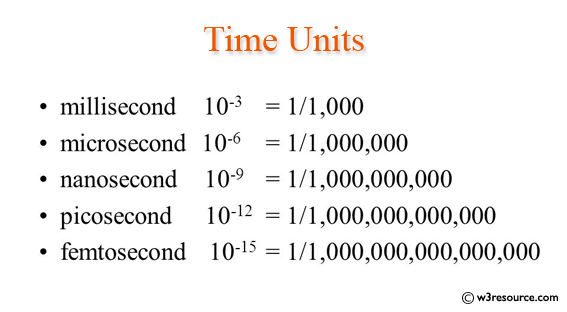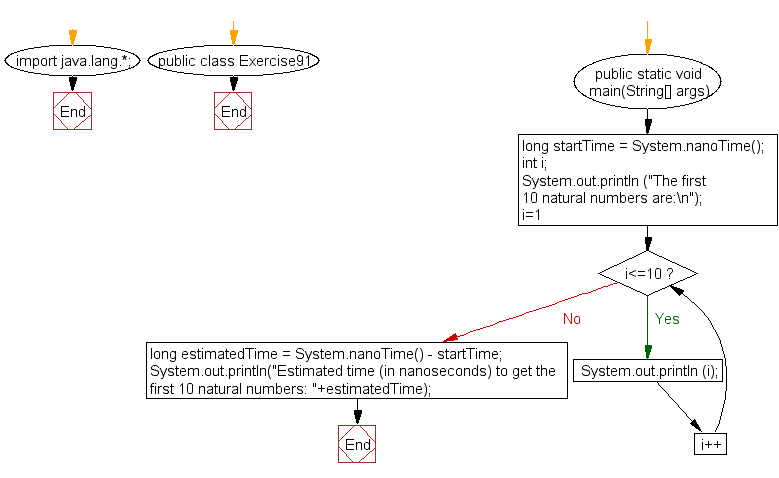﻿ Java: How long some code takes to execute in nanoseconds?# Java Exercises: Measure how long some code takes to execute in nanoseconds

## Java Basic: Exercise-91 with Solution

Write a Java program to measure how long some code takes to execute in nanoseconds.

Pictorial Presentation:Sample Solution:

Java Code:

``````import java.lang.*;
public class Exercise91 {
public static void main(String[] args)
{
long startTime = System.nanoTime();
// Sample program
// Display the first 10 natural numbers.
int i;
System.out.println ("The first 10 natural numbers are:\n");
for (i=1;i<=10;i++)
{
System.out.println (i);
}
long estimatedTime = System.nanoTime() - startTime;
System.out.println("Estimated time (in nanoseconds) to get the first 10 natural numbers: "+estimatedTime);
}
}
```
```

Sample Output:

```The first 10 natural numbers are:

1
2
3
4
5
6
7
8
9
10
Estimated time (in nanoseconds) to get the first 10 natural numbers: 29
1045
```

Flowchart:Java Code Editor:

What is the difficulty level of this exercise?

Test your Programming skills with w3resource's quiz.

﻿

## Java: Tips of the Day

countOccurrences

Counts the occurrences of a value in an array.

Use Arrays.stream().filter().count() to count total number of values that equals the specified value.

```public static long countOccurrences(int[] numbers, int value) {
return Arrays.stream(numbers)
.filter(number -> number == value)
.count();
}
```

Ref: https://bit.ly/3kCAgLb